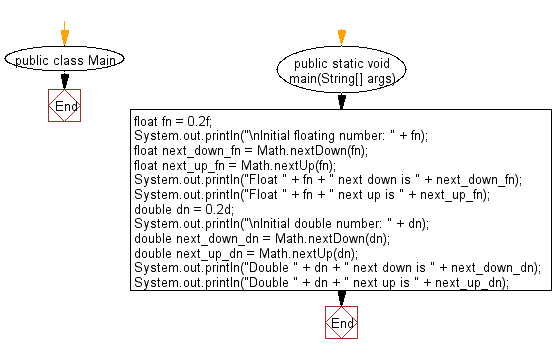﻿ Java exercises: Get the next floating-point adjacent in the direction of positive and negative infinity from a given float/double number - w3resource# Java Data Type Exercises: Get the next floating-point adjacent in the direction of positive and negative infinity from a given float/double number

## Java Data Type: Exercise-15 with Solution

Write a Java program to get the next floating-point adjacent in the direction of positive and negative infinity from a given float/double number.

Sample Solution:

Java Code:

``````public class Main {
public static void main(String[] args) {
float fn = 0.2f;
System.out.println("\nInitial floating number: " + fn);
float next_down_fn = Math.nextDown(fn);
float next_up_fn = Math.nextUp(fn);
System.out.println("Float " + fn + " next down is " + next_down_fn);
System.out.println("Float " + fn + " next up is " + next_up_fn);
double dn = 0.2d;
System.out.println("\nInitial double number: " + dn);
double next_down_dn = Math.nextDown(dn);
double next_up_dn = Math.nextUp(dn);
System.out.println("Double " + dn + " next down is " + next_down_dn);
System.out.println("Double " + dn + " next up is " + next_up_dn);
}
}
```
```

Sample Output:

```Initial floating number: 0.2
Float 0.2 next down is 0.19999999
Float 0.2 next up is 0.20000002

Initial double number: 0.2
Double 0.2 next down is 0.19999999999999998
Double 0.2 next up is 0.20000000000000004
```

Flowchart:Java Code Editor:

Improve this sample solution and post your code through Disqus

What is the difficulty level of this exercise?

﻿

## Java: Tips of the Day

Checks if a string is upper case:

```public static boolean isUpperCase(String input) {
return Objects.equals(input, input.toUpperCase());
}
```

Ref: https://bit.ly/39Hpo84